# RD Sharma Solutions Class 7 Chapter 20 Mensuration Perimeter and Area of Rectilinear Figures

Read RD Sharma Solutions Class 7 Chapter 20 Mensuration Perimeter and Area of Rectilinear Figures below, students should study RD Sharma class 7 Mathematics available on Studiestoday.com with solved questions and answers. These chapter wise answers for class 7 Mathematics have been prepared by teacher of Grade 7. These RD Sharma class 7 Solutions have been designed as per the latest NCERT syllabus for class 7 and if practiced thoroughly can help you to score good marks in standard 7 Mathematics class tests and examinations

Exercise 20.1

Question :1. Find the area, in square meters, of a rectangle whose

(i) Length = 5.5 m, breadth = 2.4 m

(ii) Length = 180 cm, breadth = 150 cm

Solution 1:

(i) Formula of area of rectangle = Length x Breadth

Length = 5.5 m, Breadth = 2.4 m

= 5.5 m x 2.4 m = 13.2 m2

(ii) Formula of area of rectangle = Length x Breadth

Length = 180 cm = 1.8 m Breadth = 150 cm = 1.5 m

Convert cm into m           (As 100 cm = 1 m)

Length = 180 ÷ 100 = 1.8 m

Breadth = 150 ÷ 100 = 1.5 m

= 1.8 m x 1.5 m = 2.7 m2

Question :2. Find the area, in square centimeters, of a square whose side is

(i) 2.6 cm

(ii) 1.2 dm

Solution 2:

(i) Formula of area of the square = (Side)2

Side of the square = 2.6 cm

= (2.6 cm)2 = (2.6 × 2.6)

= 6.76 cm2

(ii) Formula of area of the square = (Side)2

Side of the square = 1.2 dm

Convert dm into cm:

= 1.2 x 10 cm = 12 cm                      (As 1 dm = 10 cm)

= (12 cm)2 (12 × 12)

= 144 cm2

Question :3. Find in square meters, the area of a square of side 16.5 dam.

Solution  3:

Side of the square = 16.5 dam

Convert dam into m                        (As 1 dam/dm (decameter) = 10 m)

16.5 x 10 m = 165 m

Formula of area of the square = (Side)2

= (165 m)2  = 165 × 165

= 27225 m2

Question :4. Find the area of a rectangular field in acres whose sides are:

(i) 200 m and 125 m

(ii) 75 m 5 dm and 120 m

Solution 4:

(i) Length = 200 m; Breadth = 125 m

Formula of area of the rectangular = Length x Breadth

= 200 m x 125 m

= 25000 m2          (As 100 m2 = 1 acre)

= 250 acres

(ii) Length =75 m 5 dm; Breadth = 120 m

Length = (75 + 0.5) m

= 75.5 m               (As 1 dm = 10 cm = 0.1 m)

Formula of area of the rectangular = Length x Breadth

= 75.5 m x 120 m

= 9060 m2

= 90.6 acres        (As 100 m2 = 1 acre)

Question :5. Find the area of a rectangular field in hectares whose sides are:

(i) 125 m and 400 m

(ii) 75 m 5 dm and 120 m

Solution 5:

(i) Length = 125 m; Breadth = 400 m

Formula of area of the rectangular = Length x Breadth

= 125 m x 400 m

= 50000 m2

= 5 hectares       (As 10000 m2 = 1 hectare)

(ii) Length =75 m 5 dm; Breadth = 120 m

Length = (75 + 0.5) m

= 75.5 m               (As 1 dm = 10 cm = 0.1 m)

Formula of area of the rectangular field = Length x Breadth

= 75.5 m x 120 m

= 9060 m2

= 0.906 hectares               (As 10000 m2 = 1 hectare)

Question :6. A door of dimensions 3 m x 2m is on the wall of dimension 10 m x 10 m. Find the cost of painting the wall if rate of painting is Rs 2.50 per sq. m.

Solution  6:

According to the question Length of the door = 3 m, and Breadth of the door = 2 m; Side of the wall = 10 m

Formula of Area of the square = (Side)2

Area of the wall = Side x Side = 10 m x 10 m

= 100 m2

Formula of area of the rectangle = length x breadth

Area of the rectangular door = Length x Breadth

= 3 m x 2 m = 6 m

Therefore, required area of the wall for painting = Area of the wall – Area of the door

= (100 – 6) m2

= 94 m2

Rate of painting per square meter = Rs. 2.50

Hence, the cost of painting the wall = Rs. 94 x 2.50 = 235 Rs.

Question :7. A wire is in the shape of a rectangle. Its length is 40 cm and breadth is 22 cm. If the same wire is bent in the shape of a square, what will be the measure of each side. Also, find which side encloses more area?

Solution  7:

Rectangular shaped wires’ length = 40cm

Rectangular shaped wires’ breadth = 22cm

Formula of Perimeter of the rectangle = 2(Length + Breadth)

= 2(40 cm + 22 cm)

= 124 cm

It is given that the wire which was in the shape of a rectangle is now bent into a square.

Thus, the perimeter of the square = Perimeter of the rectangle

Perimeter of the square = 124 cm

Formula of perimeter of square = 4 x side

= 4 x side = 124 cm

Side = 124/4 = 31 cm

Formula of area of rectangle = length x breadth

= 40 cm x 22 cm

= 880 cm2

Formula of area of the square = (Side)2

= (31 cm)2

= 961 cm2.

So, the square-shaped wire encloses more area then a rectangular wire.

Question :8. How many square meters of glass will be required for a window, which has 12 panes, each pane measuring 25 cm by 16 cm?

Solution  8:

The glass pane’s Length = 25 cm

The glass pane’s Breadth = 16 cm

The formula of area of rectangle = length x breadth

So, the area of one glass pane = 25 cm x 16 cm

= 400 cm2

Convert cm into m           (As 1 m2 = 10000 cm2)

400 ÷ 10000

= 0.04 m2

Thus, Area of 12 panes = 12 x 0.04 = 0.48 m2

Question :9. A marble tile measures 10 cm x 12 cm. How many tiles will be required to cover a wall of size 3 m x 4 m? Also, find the total cost of the tiles at the rate of Rs 2 per tile.

Solution  9:

According to the question area of the wall = 3 m x 4 m = 12 m2

Also, area of one marble tile = 10 cm x 12 cm = 120 cm2

Convert cm into m           (As 1 m2 = 10000 c m2)

120 ÷ 10000

= 0.012 m2

Number of tiles required to cover the wall = (Area of wall)/(Area of one marble tile)

=  12/0.012 = 1000 tiles

Cost of the one tile = Rs. 2.00

Total cost = Number of tiles x Cost of one tile

= Rs. 1000 x 2 = Rs. 2000

Question :10. A table top is 9 dm 5 cm long 6 dm 5 cm broad. What will be the cost to polish it at the rate of 20 paise per square centimeter?

Solution  10:

Length of a table top = 9 dm 5 cm

Convert dm into cm        (As 1 dm = 10 cm)

= (9 x 10 + 5) cm

= 95 cm

Also, that Breadth of a table top = 6 dm 5 cm

Convert dm into cm        (As 1 dm = 10 cm)

= (6 x 10 + 5) cm

= 65 cm

Area of the rectangle (table top) = Length x Breadth

= (95 cm x 65 cm)

= 6175 c m2

Cost of polishing per square centimeter = 20 paise = Rs. 0.20 (As 1Rs. = 100 paise)

Total cost to polish table top = 6175 x 0.20

= Rs. 1235

Question :11. A room is 9.68 m long and 6.2 m wide. Its floor is to be covered with rectangular tiles of size 22 cm by 10 cm. Find the total cost of the tiles at the rate of Rs 2.50 per tile.

Solution  11:

Length of the floor of the room = 9.68 m

Breadth of the floor of the room = 6.2 m

Formula of area of rectangle = length x breadth

Area of the floor = 9.68 m x 6.2 m

= 60.016 m2

According to the question length of the tile = 22 cm;

Breadth of the tile = 10 cm

Area of one tile = 22 cm x 10 cm

= 220 cm2

Convert cm into m           (As 1 m2 = 10000 c m2)

= 220 ÷ 10000

= 0.022 m2

Thus, Number of tiles = 60.016/0.022   m2

= 2728

Cost of one tile = Rs. 2.50

Total cost = Number of tiles x Cost of one tile

= 2728 x 2.50

= Rs. 6820

Question :12. One side of a square field is 179 m. Find the cost of raising a lawn on the field at the rate of Rs 1.50 per square meter.

Solution  12:

One side of the square field = 179 m

Area of the square field = Side 2

= 179 m 2 = 179 × 179

= 32041 m2

The price of mowing a lawn on a field per square meter = Rs. 1.50

Thus, total cost of raising a lawn on the field

= 32041 x 1.50 = Rs. 48061.50

Question :13. A rectangular field is measured 290 m by 210 m. How long will it take for a girl to go two times round the field, if she walks at the rate of 1.5 m/sec?

Solution  13:

Length of the rectangular field = 290 m

Also, Breadth of the rectangular field = 210 m

Formula of perimeter of the rectangular field = 2(Length + Breadth)

= 2(290 + 210) = 1000 m

Here we calculate distance covered by the girl = 2 x Perimeter of the rectangular field

= 2 x 1000 = 2000 m

According to the question the girl walks at the rate of 1.5 m/sec;

Speed of the girl = 1.5 x 60 m/min = 90 m/min

Speed = Distance/ Time

Hence, time taken by the girl = Distance/Speed

= 2000/90

= 22 2/9  min

Hence, the girl will take

= 22 2/9 min to go two times around the field.

Question :14. A corridor of a school is 8 m long and 6 m wide. It is to be covered with canvas sheets. If the available canvas sheets have the size 2 m x 1 m, find the cost of canvas sheets required to cover the corridor at the rate of Rs 8 per sheet.

Solution  14:

Length of the corridor = 8 m

Also, breadth of the corridor = 6 m

Area of the corridor of a school = Length x Breadth

= 8 x 6 = 48 m2

The canvas sheets’ length = 2 m

The canvas sheets’ breadth = 1 m

Area of one canvas sheet = Length x Breadth

= 2 m x 1 m = 2 m2

Thus, Canvas sheets required to cover the corridor =  48/2   = 24 Sheets

According to the question cost of one canvas sheet = Rs. 8

Total cost of the canvas sheets = number of canvas sheet x cost of one canvas sheet

= (24 x 8) = Rs. 192

Question :15. The length and breadth of a playground are 62 m 60 cm and 25 m 40 cm respectively. Find the cost of turfing it at Rs 2.50 per square meter. How long will a man take to go three times round the field, if he walks at the rate of 2 meters per second?

Solution  15:

Length of playground = 62 m 60 cm

Convert cm into m           (As 10 cm = 0.1 m)

= 62.6 m

Breadth of a playground = 25 m 40 cm

Convert cm into m            (As 10 cm = 0.1 m)

= 25.4 m

Area of a rectangular playground = Length x Breadth

= 62.6 m x 25.4 m

= 1590.04 m2

According to the question rate of turfing

= Rs. 2.50/ m2

The total cost of the turfing

= 1590.04 x 2.50

= Rs. 3975.10

Perimeter of a rectangular field = 2(Length + Breadth)

= 2(62.6 + 25.4) = 176 m

Distance covered by the man in 3 rounds of a field = 3 x Perimeter of a rectangular field

= 3 x 176 m = 528 m

The man walks at the rate of 2 m/sec.

Speed of man = 2 x 60 m/min = 120 m/min

Thus, time to cover a distance of 528 m = 528/120

= 4.4 min

Therefore, 4 minutes 24 seconds

Question :16. A lane 180 m long and 5 m wide is to be paved with bricks of length 20 cm and breadth 15 cm. Find the cost of bricks that are required, at the rate of Rs 750 per thousand.

Solution  16:

Length of lane = 180 m

Breadth of lane = 5 m

Area of a rectangular lane = Length x Breadth

= 180 m x 5 m

= 900 m2

Length of brick = 20 cm

Breadth of brick = 15 cm

Area of a rectangular brick = Length x Breadth

= 20 cm x 15 cm

= 300 cm2

Convert cm into m           (As 1 m2 = 10000 cm2)

= 0.03 m2

Number of bricks =  900/0.03  = 30000

Cost of 1000 bricks = Rs. 750

Total cost of 30,000 bricks =  (750 x 30000)/1000

= Rs. 22,500

Question :17. How many envelopes can be made out of a sheet of paper 125 cm by 85 cm; supposing one envelope requires a piece of paper of size 17 cm by 5 cm?

Solution  17:

Given in the question Length of paper sheet = 125 cm

Breadth of the paper sheet = 85 cm

Area of a rectangular paper sheet = Length x Breadth

= 125 cm x 85 cm

= 10,625 cm2

Length of sheet needed for an envelope = 17 cm

Breadth of sheet needed for an envelope = 5 cm

Area of the sheet needed for a single envelope = Length x Breadth

= 17 cm x 5 cm

= 85 c m2

Thus, number of envelopes =  10,625/85

= 125

Question :18. The width of a cloth is 170 cm. Calculate the length of the cloth required to make 25 diapers, if each diaper requires a piece of cloth of size 50 cm by 17 cm.

Solution  18:

Length of the diaper = 50 cm

Breadth of the diaper = 17 cm

Area of cloth to make 1 diaper = Length x Breadth

= 50 cm x 17 cm

= 850 cm2

Thus, Area of 25 diapers

= 25 x 850 cm2

= 21,250 cm2

Area of total cloth for 25 diapers

= Area of 25 diapers = 21,250 cm2

According to the question that width of a cloth = 170 cm

Length of the cloth = Area of cloth

Width of cloth = 170cm

Length of a cloth = 21,250/170 cm = 125 cm

So, the length of cloth will be 125 cm.

Question :19. The carpet for a room 6.6 m by 5.6 m costs Rs 3960 and it was made from a roll 70 cm wide. Find the cost of the carpet per meter.

Solution  19:

Length of the carpet for a room = 6.6 m

Breadth of the carpet for a room = 5.6 m

Area of a rectangular room = Length x Breadth

= 6.6 m x 5.6 m

= 36.96 m2

Width of a carpet = 70 cm

Convert cm into m           (As 1 m = 100 cm)

= 0.7 m

Length of a carpet x Width of a carpet = Area of a room

36.96 = Length of the carpet × 0.7

Length of the carpet =  36.96/0.7

= 52.8 m

Cost of the carpet per meter = (Total cost of the carpet)/(Length of the carpet)

= 3960/52.8

= Rs. 75

Question :20. A room is 9 m long, 8 m broad and 6.5 m high. It has one door of dimensions 2 m x 1.5 m and three windows each of dimensions 1.5 m x 1 m. Find the cost of white washing the walls at Rs 3.80 per square meter.

Solution  20:

According to the question given information are Length of a room = 9 m

Breadth of a room = 8 m

Height of a room = 6.5 m

Area of 4 walls = 2(l + b) h

= 2 (9 m + 8 m) x 6.5 m

= 2 x 17 m x 6.5 m

= 221 m2

Length of a door = 2 m

Breadth of a door = 1.5 m

Formula of area of a rectangular door = Length x Breadth

= 2 m x 1.5 m

= 3 m2

Length of a window = 1.5 m

Breadth of a window = 1 m

Formula of area of one window = Length x Breadth

= 1.5 m x 1 m

= 1.5 m2

Thus, Area of 3 such windows

= 3 x 1.5 m2

= 4.5 m2

Area to be white washed = Area of 4 walls – (Area of one door + Area of 3 windows)

Area to be white washed = [221 – (3 + 4.5)] m2

= 221 – 7.5 m2

= 213.5 m2

Cost of white washing for 1 m2 area = Rs. 3.80

Cost of white washing for 213.5 m2 area = Rs. 213.5 x 3.80

= Rs. 811.30

Question :21. A hall 36 m long and 24 m broad allowing 80 m2 for doors and windows, the cost of papering the walls at Rs 8.40 per m2 is Rs 9408. Find the height of the hall.

Solution  21:

Length of the hall = 36 m

Breadth of the hall = 24 m

Let i be the height of the hall.

Now, we must paper the four walls of the chamber, except the floor and roof. As a result, the area of the wall to be papered equals the = Area of 4 walls

= 2 i (I + b)

= 2 i (36 + 24)

= 120 im2

Now there's just enough space for the door and windows = 80 m2

As a result, the region that is currently papered = (120 i – 80) m2

Cost of papering the wall = Area required for papering × Cost per meter square of papering wall

9408 = (120 i – 80) × 8.40

1120 = 120 i – 80

1200 = 120 i

i = 10 m

So, the height of the wall would be 10 m.

Exercise 20.2

Question :1. A rectangular grassy lawn measuring 40 m by 25 m is to be surrounded externally by a path which is 2 m wide. Calculate the cost of leveling the path at the rate of Rs 8.25 per square meter.

Solution  1: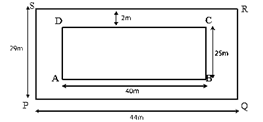Let PQRS be the rectangular grassy lawn

Let length = AB

According to question length PQ = 40 m and breadth QR = 25 m

Area of rectangular lawn PQRS = 40 m x 25 m = 1000 m2

Length of AB = 40 + 2 + 2 m

= 44 m

Breadth of BC = 25 + 2 + 2 m

= 29 m

Area of ABCD = 44 m x 29 m

= 1276 m2

Now, Area of the path = Area of ABCD – Area of the lawn PQRS

= 1276 m2 – 1000 m2

= 276 m2

Rate of levelling path

= Rs. 8.25 per m2

Cost of levelling path

= 8.25 x 276

= Rs. 2277

Question :2. One-meter-wide path is built inside a square park of side 30 m along its sides. The remaining part of the park is covered by grass. If the total cost of covering by grass is Rs 1176, find the rate per square meter at which the park is covered by the grass.

Solution 2:Side of a square garden = 30m = a

Formula of the area of square = a2

Area of the square garden including the path = 302

30 × 30 = 900 m2

According to the figure, When the path is not included, the side of the square garden can be seen is = 28 m.

Area of the square garden not including the path = 28 2

28 × 28 = 784 m2

Total cost of covering the park with grass = Area of the park covering with green grass x Rate per sq. me

1176 = 784 x Rate per square meter

Rate per sq. m at which the park is covered with grass = (1176/784)

= Rs. 1.50 per m2

Question :3. Through a rectangular field of sides 90 m x 60 m, two roads are constructed which are parallel to the sides and cut each other at right angles through the center of the field. If the width of the roads is 3 m, find the total area covered by the two roads.

Solution 3:Rectangular fields’ length = 90m

Area of the rectangular field = 90 m x 60 m

= 5400 m2

According to the question length = 90 m and breadth = 3m

= 90 m x 3 m

= 270 m2

According to the question length = 90 m and breadth = 3m

= 60 m x 3 m

= 180 m2

So, area of KLMN is common to the two roads.

Thus, area of KLMN = 3 m x 3 m

= 9 m2

Hence, area of the roads = Area (PQRS) + Area (ABCD) – Area (KLMN)

= (270 + 180) m2 – 9 m2

= 441 m2

Question :4. From a rectangular sheet of tin, of size 100 cm by 80 cm, are cut four squares of side 10 cm from each corner. Find the area of the remaining sheet.

Solution  4: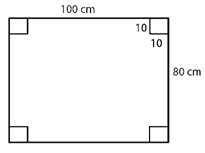Length of rectangular sheet = 100 cm; Breadth of rectangular sheet = 80 cm

Area of the rectangular tin sheet = 100 cm x 80 cm

= 8000 c m2

Area of one square at the corner of the sheet = 10 cm2

= 100 cm2

Area of 4 squares at the corner of the sheet = 4 x 100 cm2

= 400 cm2

Hence, Area of the remaining tin sheet = Area of the rectangular sheet – Area of the 4 squares

Area of the remaining tin sheet = 8000 – 400 cm2

= 7600 cm2

Question :5. A painting 8 cm long and 5 cm wide is painted on a cardboard such that there is a margin of 1.5 cm along each of its sides. Find the total area of the margin.

Solution  5:Length of cardboard with margin = 8 cm

Breadth of the cardboard with margin = 5 cm

Area of the cardboard with the margin

= 8 cm x 5 cm = 40 c m2

According to the diagram,

New length of the painting excluded margin

= 8 cm – (1.5 cm + 1.5 cm)

= (8 – 3) cm

= 5 cm

New breadth of the painting excluded margin

= 5 cm – (1.5 cm + 1.5 cm)

= (5 – 3) cm

= 2 cm

Area of the painting without the margin = 5 cm x 2 cm = 10 cm2

Hence, Area of the margin = Area of the cardboard with the margin – Area of the painting

= (40 – 10) cm2

= 30 cm2

Question :6. Rakesh has a rectangular field of length 80 m and breadth 60 m. In it, he wants to make a garden 10 m long and 4 m broad at one of the corners and at another corner, he wants to grow flowers in two floor-beds each of size 4 m by 1.5 m. In the remaining part of the field, he wants to apply manures. Find the cost of applying the manures at the rate of Rs 300 per area.

Solution  6: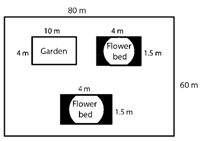Length of a rectangular field = 80 m

Breadth of a rectangular field = 60 m

Area of rectangular field = length x breadth

= (80 x 60) m

= 4800 m2

Area of the garden = 10 m x 4 m

= 40 m2

Area of 1 flower bed = 4 m x 1.5 m

= 6 m2

Area of 2 flower beds = 2 x 6 m2 = 12 m2

Remaining area of the field = Area of the rectangular field – (Area of the garden + Area of the 2 flower beds)

Remaining area of the field = 4800 m2 – (40 + 12) m2

= (4800 – 52) m2

= 4748 m2

4748 m2 = 47.48 acres                     (As 100 m2 = 1 acre)

So, The cost of applying manure at the rate of Rs. 300 per area will be = Rs. (300 x 47.48)

= Rs. 14244

Question :7. Each side of a square flower bed is 2 m 80 cm long. It is extended by digging a strip 30 cm wide all around it. Find the area of the enlarged flower bed and also the increase in the area of the flower bed.

Solution  7: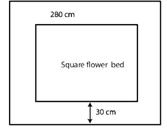Side of the flower bed = 2 m 80 cm

= 2.80 m               (As 100cm = 1m)

Area of the square flower bed = (Side)2

= 2.80 m2

= 7.84 m2

Side of the flower bed included strip = 2.80 m + 30 cm + 30 cm

= 2.80 + 0.3 + 0.3 m

= 3.4 m

Area of the enlarged flower bed included the digging strip = Side2

= 3.42

= 11.56 m2

Thus, Growth in the area of the flower bed = Area of the enlarged flower bed with the digging strip – Area of the square flower bed

= 11.56 m2 – 7.84 m2

= 3.72 m2

Question :8. A room 5 m long and 4 m wide is surrounded by a verandah. If the verandah occupies an area of 22 m2, find the width of the verandah.

Solution  8:Let the x m be width of the verandah.

Length of the room AB = 5 m and breadth of the room, BC = 4 m

Area of rectangle = length x breadth

Area of the room = 5 m x 4 m

= 20 m2

According to the diagram,

Length of the veranda PQ

= (5 + x + x) = (5 + 2x) m

= (4 + x + x) = (4 + 2x) m

Area of veranda PQRS

= (5 + 2x) x (4 + 2x)

= (4×2 + 18x + 20) m2

Area of veranda = Area of PQRS – Area of ABCD

22 = 4x2 + 18x + 20 – 20

22 = 4x2 + 18x

By dividing above equation by 2,

11 = 2x2 + 9x

2x2 + 9x – 11 = 0

2x2 + 11x – 2x – 11 = 0

x (2x+11) – 1 (2x+11) = 0

(x- 1) (2x+11) = 0

When x – 1 = 0, x = 1

When 2x + 11 = 0, x = (-11/2)

The width cannot be a negative value.

So, width of the veranda = x = 1 m

Question :9. A square lawn has a 2 m wide path surrounding it. If the area of the path is 136 m2, find the area of the lawn.

Solution  9:Let ABCD be the square lawn and PQRS be the outer boundary of the square path.

Let x m be the side of the lawn AB.

Area of the square lawn = x2

According to the question length PQ

= (x + 2 + 2) = (x + 4) m

Area of PQRS = (x + 4)2 = (x2 + 8x + 16) m2

Now, Area of the path = Area of PQRS – Area of the square lawn

136 = x2 + 8x + 16 – x2

136 = 8x + 16

136 – 16 = 8x

120 = 8x

x =  120/8  = 15

Side of the lawn = 15 m

So, Area of the lawn = Side2

= 15 m2

= 225 m2

Question :10. A poster of size 10 cm by 8 cm is pasted on a sheet of cardboard such that there is a margin of width 1.75 cm along each side of the poster. Find (i) the total area of the margin (ii) the cost of the cardboard used at the rate of Rs 0.60 per c m2.

Solution  10: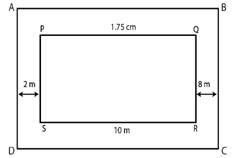According to the question length of poster = 10 cm and breadth of poster = 8 cm

Area of the poster = Length x Breadth

= 10 cm x 8 cm

= 80 cm2

According to the diagram,

Length of the cardboard included the margin = 10 cm + 1.75 cm + 1.75 cm

= 13.5 cm

Breadth of the cardboard included the margin = 8 cm + 1.75 cm + 1.75 cm

= 11.5 cm

Area of the cardboard = Length x Breadth

= 13.5 cm x 11.5 cm

= 155.25 c m2

(i) Area of the margin = Area of cardboard with the margin – Area of the poster

= 155.25 c m2 – 80 c m2

= 75.25 c m2

(ii) Cost of the cardboard = Area of cardboard x Rate of the cardboard Rs 0.60 per cm2

= (155.25 x 0.60)

= Rs. 93.15

Question :11. A rectangular field is 50 m by 40 m. It has two roads through its center, running parallel to its sides. The widths of the longer and shorter roads are 1.8 m and 2.5 m respectively. Find the area of the roads and the area of the remaining portion of the field.

Solution 11: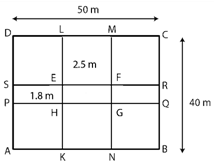Let ABCD be the rectangular field

Width of KLMN rectangular road = 1.8 m and width of the PQRS rectangular road= 2.5 m.

Length of rectangular field = 50 m

Breadth of rectangular field = 40m

Area of rectangular field ABCD

= 50 m x 40 m

= 2000 m2

= 40 m x 2.5 m

= 100 m2

= 50 m x 1.8 m

= 90 m2

Area of EFGH is common to the two roads.

Thus, Area of EFGH = 2.5 m x 1.8 m = 4.5 m2

So, Area of the roads = Area of KLMN + Area of PQRS – Area of EFGH

= (100 m2 + 90 m2) – 4.5 m2

= 185.5 m2

Area of the enduring portion of the field = Area of the rectangular field ABCD – Area of the roads

= 2000 – 185.5 m2

= 1814.5 m2

Question :12. There is a rectangular field of size 94 m x 32 m. Three roads each of 2 m width pass through the field such that two roads are parallel to the breadth of the field and the third is parallel to the length. Calculate: (i) area of the field covered by the three roads (ii) area of the field not covered by the roads.

Solution  12: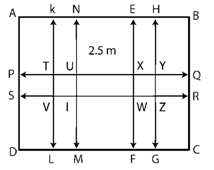Let ABCD be the rectangular field.

From the figure it is clear that two roads which are parallel to the breadth of the field KLMN and EFGH with width 2 m each.

One road which is parallel to the length of the field PQRS with width 2 m.

We have from the question, length of the rectangular field AB = 94 m and breadth of the rectangular field BC = 32 m

Area of the rectangular field = Length x Breadth

= 94 m x 32 m

= 3008 m2

= 32 m x 2 m

= 64 m2

= 32 m x 2 m

= 64 m2

= 94 m x 2 m

= 188 m2

Area of VTUI and WXYZ is common to these three roads.

Thus, Area of VTUI

= 2 m x 2 m = 4 m2

Area of WXYZ

= 2 m x 2 m = 4 m2

(i) Area of the field covered by the three roads = Area of KLMN + Area of EFGH + Area (PQRS) – (Area of VTUI + Area of WXYZ)

= [64+ 64 + 188 – (4 + 4)] m2

= 316 m2 – 8 m2

= 308 m2

(ii) Area of the field not covered by the roads = Area of the rectangular field ABCD – Area of the field covered by the three roads

= 3008 m2 – 308 m2

= 2700 m2

Question :13. A school has a hall which is 22 m long and 15.5 m broad. A carpet is laid inside the hall leaving all around a margin of 75 cm from the walls. Find the area of the carpet and the area of the strip left uncovered. If the width of the carpet is 82 cm, find the cost at the rate of Rs 18 per meter.

Solution  13:Length of hall PQ = 22 m and breadth of hall QR = 15.5 m

Area of the school hall PQRS = 22 m x 15.5 m

= 341 m2

Length of the carpet AB

= 22 m – (0.75 m + 0.75 m)

= 20.5 m

= 15.5 m – (0.75 m + 0.75 m)

= 14 m

Area of the carpet ABCD

= 20.5 m x 14 m

= 287 m2

Area of the strip = Area of the school hall PQRS – Area of the carpet ABCD

= 341 m2 – 287 m2

= 54 m2

Now, area of 1 m length of carpet = 1 m x 0.82 m

= 0.82 m2

Thus, Length of carpet whose area is 287 m2 = 287 m+ 0.82 m2

= 287.82 m

Cost of the 287.82 m long carpet = Rs. 18 x 287.82

= Rs. 5180.76

Question :14. Two cross roads, each of width 5 m, run at right angles through the center of a rectangular park of length 70 m and breadth 45 m parallel to its sides. Find the area of the roads. Also, find the cost of constructing the roads at the rate of Rs 105 per m2.

Solution 14: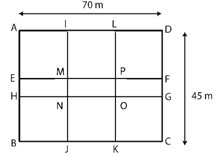Let ABCD be the rectangular park then EFGH and IJKL are the two rectangular roads with width 5m.

According to the question length of rectangular park = 70m and breadth of rectangular park = 45m

Area of the rectangular park = Length x Breadth

= 70 m x 45 m

= 3150 m2

= 70 m x 5 m

= 350 m2

Now, Area of the road JKLI

= 45 m x 5 m

= 225 m2

According to the diagram area of MNOP is common to the two roads.

Thus, Area of MNOP

= 5 m x 5 m = 25 m2

Thus, Area of the roads = Area of EFGH + Area of JKLI – Area of MNOP

= 350 m2 + 225 m2– 25 m2

= 550 m2

The cost of constructing the roads = Rs. 105 per m2

Thus, Cost of constructing 550 m2 area of the roads

= Rs. (105 x 550)

= Rs. 57750.

Question :15. The length and breadth of a rectangular park are in the ratio 5: 2. A 2.5 m wide path running all around the outside the park has an area 305 m2. Find the dimensions of the park.

Solution 15: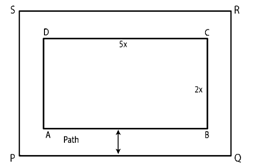Area of the park be 305m2

Let the length of the park be 5x m and breadth be 2x m.

Thus, area of the rectangular park = (5x) x (2x)

= 10x2 m2

Width of the path = 2.5 m

According to the diagram, External length PQ

= 5x m + 2.5 m + 2.5 m

= (5x + 5) m

External breadth QR = 2x + 2.5 m + 2.5 m

= 2x + 5 m

Area of PQRS

= (5x + 5) m x (2x + 5) m

= (10x2 + 25x + 10x + 25) m2

= (10x2 + 35x + 25) m2

Area of the path

= [(10x2 + 35x + 25) – 10x2] m2

By solving above equation,

305 = 35x + 25

305 – 25 = 35x

280 = 35x

x =  280/35   = 8

Thus, length of the park = 5x

= 5 x 8 = 40 m

And, Breadth of the park = 2x

= 2 x 8 = 16 m

Question :16. A square lawn is surrounded by a path 2.5 m wide. If the area of the path is 165 m2, find the area of the lawn.

Solution  16:Let x m be the side of lawn.

According to the question width of the path = 2.5 m

Side of the lawn with the path

= (x + 2.5 + 2.5) m

= (x + 5) m

Thus, area of lawn = (Area of the lawn with the path) – (Area of the path)

Formula of the area of a square = (Side)2

Area of lawn (x2)

= (x + 5)2 – 165

x2 = (x2 + 10x + 25) – 165

165 = 10x + 25

165 – 25 =10x

140 = 10x

Thus, x =     = 14

Thus, the side of the lawn = 14 m

The area of the lawn = (14 m) 2

= 196 m2

Exercise 20.3

Question :1. Find the area of a parallelogram with base 8 cm and altitude 4.5 cm.

Solution  1:

Base = 8 cm and altitude = 4.5 cm

Formula of the area of the parallelogram = Base x Altitude

= 8 cm x 4.5 cm

So, area of parallelogram = 36 cm2

Question :2. Find the area in square meters of the parallelogram whose base and altitudes are as under

(i) Base =15 dm, altitude = 6.4 dm

(ii) Base =1 m 40 cm, altitude = 60 cm

Solution 2:

(i) Base =15 dm, altitude = 6.4 dm

To convert dm into m, first we convert them cm:

Base = 15 dm

= (15 x 10) cm = 150 cm = 1.5 m

Altitude = 6.4 dm

= (6.4 x 10) cm = 64 cm = 0.64 m

Formula of area of the parallelogram = Base x Altitude

= 1.5 m x 0.64 m

Area of parallelogram = 0.96 m2

(ii) Base =1 m 40 cm, altitude = 60 cm

Convert cm into m           (As 100 cm = 1 m)

Base = 1 m 40 cm = 1.4 m

Altitude = 60 cm = 0.6 m

Formula of the area of the parallelogram = Base x Altitude

= 1.4 m x 0.6 m

= 0.84 m2

Question :3.  Find the altitude of a parallelogram whose area is 54 d m2 and base is 12 dm.

Solution  3:

Area of the parallelogram = 54 d m2

Base of the parallelogram = 12 dm

Formula of the area of the parallelogram = Base x Altitude

Thus, altitude of the parallelogram = Area/Base

=   54/12 dm = 4.5 dm

Question :4. The area of a rhombus is 28 m2. If its perimeter be 28 m, find its altitude.

Solution  4:

Perimeter of a rhombus = 28 m

As the perimeter of a rhombus = 4 (Side)

4(Side) = 28 m

Side =  28/4

Side = 7m

Now, Area of the rhombus = 28 m2

Formula of the area of rhombus = Side x Altitude

(Side x Altitude) = 28 m2

(7 x Altitude) = 28 m2

Altitude =  28/4 = 4 m

Question :5. In Fig. 20, ABCD is a parallelogram, DL ⊥ AB and DM ⊥ BC. If AB = 18 cm, BC =12 cm and DM= 9.3 cm, find DL.Solution 5:

According to the question DL ⊥ AB and DM ⊥ BC

By taking BC as the base, BC = 12 cm

By taking DM as the altitude = 9.3 cm

Formula of the area of parallelogram ABCD = Base x Altitude

= 12 cm x 9.3 cm

= 111.6 c m2 - Equation (i)

Now, by taking AB as the base,

Area of the parallelogram ABCD = Base x Altitude

= 18 cm x DL - Equation (ii)

By Equation (i) and (ii),

18 cm x DL = 111.6 c m2

DL =  111.6/18

= 6.2 cm

Question :6. The longer side of a parallelogram is 54 cm and the corresponding altitude is 16 cm. If the altitude corresponding to the shorter side is 24 cm, find the length of the shorter side.

Solution  6: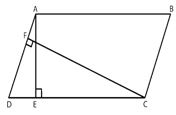Let ABCD is a parallelogram with the longer side AB = 54 cm

corresponding altitude AE = 16 cm.

The shorter side is BC

The corresponding altitude is CF = 24 cm.

Formula of the area of a parallelogram = base x height.

Two altitudes and two corresponding bases.

By equating them, = ½ x BC x CF = ½ x AB x AE

On simplifying, BC x CF = AB x AE

BC x 24 = 54 x 16

BC =    (54 x 16)/24

= 36 cm

So, the length of shorter side BC = AD = 36 cm.

Question :7. In Fig. 21, ABCD is a parallelogram, DL ⊥ AB. If AB = 20 cm, AD = 13 cm and area of the parallelogram is 100 c m2, find AL.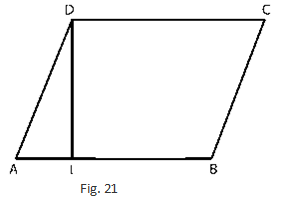Solution 7:

According to the diagram ABCD is a parallelogram with base AB = 20 cm and corresponding altitude DL.

The area of the parallelogram ABCD = 100 c m2

The area of a parallelogram = Base x Height

Thus,

100 = AB x DL

100 = 20 x DL

DL = 100/20 = 5 cm

By apply the Pythagoras theorem,

(13)2 = (AL)2+ (5)2

(AL)2 = (13)2 – (5)2

(AL)2 = 169 – 25

= 144

So, 12= 144

(AL)2 = (12)2

AL = 12 cm

So, length of AL is 12 cm.

Question :8. In Fig. 21, if AB = 35 cm, AD= 20 cm and area of the parallelogram is 560 cm2, find LB.Solution 8:

According to the diagram, ABCD is a parallelogram with base AB = 35 cm and corresponding altitude DL.

According to the question the area of the parallelogram ABCD = 560 cm2

Formula of the area of the parallelogram = Base x Height

560 cm2 = AB x DL

560 cm2 = 35 cm x DL

DL = 560/35

= 16 cm

By Pythagoras theorem,

(20)2= (AL)2 + (16)2

(AL)= (20)– (16)2

= 400 – 256

= 144

(AL)2 = (12)2

AL = 12 cm

According to the diagram, AB = AL + LB

35 = 12 + LB

LB = 35 – 12 = 23 cm

So, length of LB is 23 cm.

Question :9. The adjacent sides of a parallelogram are 10 m and 8 m. If the distance between the longer sides is 4 m, find the distance between the shorter sides

Solution  9: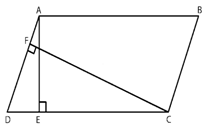Let ABCD is a parallelogram with side AB = 10 m and corresponding altitude AE = 4 m.

The adjacent side AD = 8 m and the corresponding altitude is CF.

Formula of area of a parallelogram = Base x Height

Two altitudes and two corresponding bases.

So, equating them, AD x CF = AB x AE

8 x CF = 10 x 4

CF = (10x4)8 = 5 m

So, the distance between the shorter sides is 5 m.

Question :10. The base of a parallelogram is twice its height. If the area of the parallelogram is 512 cm2, find the base and height.

Solution  10:

Let x cm the height of the parallelogram.

According to the question the base of the parallelogram is 2x cm,

The area of the parallelogram = 512 cm2

Formula of the area of a parallelogram = Base x Height

512 = (2x) (x)

512 = 2x2

x= 512/2

= 256 cm2

x2 = (16)2

x = 16 cm

So, height of parallelogram = x = 16cm

And base of the parallelogram = 2x = 2 x 16 = 32 cm

Question :11. Find the area of a rhombus having each side equal to 15 cm and one of whose diagonals is 24 cm.

Solution  11:Let ABCD be the rhombus where diagonals intersect at 0.

Then AB = 15 cm and AC = 24 cm.

The diagonals of a rhombus bisect each other at right angles.

Thus, according to the diagram triangle A0B is a right-angled triangle, with name O.

Thus, OA = ½(AC) = ½ (24) = 12 cm and AB = 15 cm.

By applying Pythagoras theorem,

(AB)2 = (OA)2 + (OB)2

(15)2 = (12)2 + (OB)2

(OB)2= (15)2– (12)2

(OB)= 225 – 144

= 81

(OB)2 = (9)2

OB = 9 cm

BD = 2 x OB

= 2 x 9

= 18 cm

So, Area of the rhombus ABCD = (½ x AC x BD)

= ( 1/2 x 24 x 18)

= 216 cm2

Question :12. Find the area of a rhombus, each side of which measures 20 cm and one of whose diagonals is 24 cm.

Solution  12:Let ABCD be the rhombus whose diagonals intersect at 0.

Then AB = 20 cm and AC = 24 cm

The diagonals of a rhombus bisect each other at right angles.

Thus, Triangle AOB is a right-angled triangle, with name O.

OA = 1/2 (AC) =  1/2 (24) = 12 cm and AB =20 cm

By applying Pythagoras theorem,

(AB)2 = (OA)2+ (OB) 2

(20)2 = (12)2 + (OB) 2

(OB) 2= (20) 2– (12) 2

(OB)= 400 – 144

= 256

(OB) 2 = (16) 2

OB = 16 cm

BD =2 x OB

= 2 x 16 cm

= 32 cm

So, area of the rhombus ABCD = 1/2 x AC x BD

= ½ x 24 x 32

Area of rhombus ABCD = 384 cm2

Question :13. The length of a side of a square field is 4 m. What will be the altitude of the rhombus, if the area of the rhombus is equal to the square field and one of its diagonals is 2 m?

Solution  13: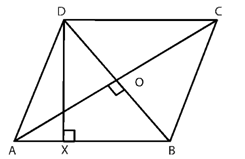Length of a side of a square field is 4m

Area of the rhombus = Area of the square of side

1/2 x AC x BD = (4m)2

1/2 x AC x 2 = 16 m2

AC = 16 m2

The diagonals of a rhombus are perpendicular bisectors of each other.

AO = 1/2 (AC) =  1/2(16) = 8 m and BO =  1/2(BD) =  1/2(2) = 1 m

By applying Pythagoras theorem,

AO2 + BO2 = AB2

AB2 = (8 m) 2 + (1 m) 2

= 64 m2 + 1 m2 = 65 m2

Side of a rhombus = AB = √65 m.

Let DX be the altitude.

Area of the rhombus = AB x DX

16 m2 = √65m × DX

DX =  16/ (√65)m

So, the altitude of the rhombus will be  16/ (√65) m.

Question :14. Two sides of a parallelogram are 20 cm and 25 cm. If the altitude corresponding to the sides of length 25 cm is 10 cm, find the altitude corresponding to the other pair of sides.

Solution  14: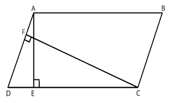Let ABCD is a parallelogram with longer side AB = 25 cm and altitude AE = 10 cm.

Thus, AB = CD because opposite sides of parallelogram are equal.

The shorter side is AD = 20 cm and the corresponding altitude is CF.

Formula of area of a parallelogram = Base x Height

The two altitudes and two corresponding bases.

So, by equating them

AD x CF = CD x AE

20 x CF = 25 x 10

CF = 12.5 cm

So, the altitude corresponding to the other pair of the side AD is 12.5 cm.

Question :15. The base and corresponding altitude of a parallelogram are 10 cm and 12 cm respectively. If the other altitude is 8 cm, find the length of the other pair of parallel sides.

Solution  15:Let ABCD is a parallelogram with side AB = CD = 10 cm because opposite sides of parallelogram are equal and corresponding altitude AM = 12 cm.

The other side is AD and the corresponding altitude is CN = 8 cm.

Formula of the area of a parallelogram = Base x Height

The two altitudes and two corresponding bases.

So, by equating them

AD x CN = CD x AM

AD x 8 = 10 x 12

AD = (10 x 12)/8 = 15 cm

So, the length of the other pair of the parallel sides = 15 cm.

Question :16. A floral design on the floor of a building consists of 280 tiles. Each tile is in the shape of a parallelogram of altitude 3 cm and base 5 cm. Find the cost of polishing the design at the rate of 50 paise per cm2.

Solution  16:

Altitude of a tile = 3 cm

Base of a tile = 5 cm

Area of one tile = Attitude x Base

= 5 cm x 3 cm

= 15 c m2

Area of 280 tiles

= 280 x 15 c m2

= 4200 c m2

Rate of polishing the tile = Rs. 0.5 per cm2

Thus, Total cost of polishing the design

= 4200 x 0.5

= Rs. 2100

Exercise 20.4

Question :1. Find the area in square centimeters of a triangle whose base and altitude are as under:

(i) Base =18 cm, altitude = 3.5 cm

(ii) Base = 8 dm, altitude =15 cm

Solution 1:

(i) Base = 18 cm and height = 3.5 cm

Formula of the area of a triangle =  1/2(Base x Height)

Thus, area of the triangle =  1/2 x 18 x 3.5

= 31.5 cm2

(ii) Base = 8 dm and height = 15 cm

Convert base = 8 dm = (8 x 10) cm = 80 cm            (As 1 dm = 10 cm)

Formula of the area of a triangle =  1/2(Base x Height)

Thus, area of the triangle = 1/2 x 80 x 15

= 600 cm2

Question :2. Find the altitude of a triangle whose area is 42 cm2 and base is 12 cm.

Solution  2:

Base = 12 cm and area = 42 cm2

Formula of the area of a triangle =  1/2(Base x Height)

Thus, altitude of a triangle = (2xArea)/Base

Altitude = (2x42)/12

= 7 cm

Question :3. The area of a triangle is 50 cm2. If the altitude is 8 cm, what is its base?

Solution  3:

Altitude = 8 cm and area = 50 cm2

Formula of the area of a triangle =  1/2(Base x Height)

Thus, base of a triangle = (2xArea)/Altitude

Base = (2x50)/8

= 12.5 cm

Question :4. Find the area of a right-angled triangle whose sides containing the right angle are of lengths 20.8 m and 14.7 m.
Solution  4:
In a right-angled triangle,
The sides containing the right angles are of lengths 20.8 m and 14.7 m.
Let the base be 20.8 m and the height be 14.7 m.
Then, formula of the area of a triangle = 1/2 (Base x Height)
= 1/2 (20.8 × 14.7)
= 152.88 m2

Question :5. The area of a triangle, whose base and the corresponding altitude are 15 cm and 7 cm, is equal to area of a right triangle whose one of the sides containing the right angle is 10.5 cm. Find the other side of this triangle.
Solution   5:
According to the question Base = 15 cm and altitude = 7 cm
Formula of the area of a triangle = 1/2 (Base x Altitude)
= 1/2 (15 x 7)
= 52.5 cm2
By the question Area of the first triangle and the second triangle are equal.
Area of the second triangle = 52.5 c m2
One side of the second triangle = 10.5 cm
Thus, the other side of the second triangle = ((2 x Area))/(One side of a triangle)
=  ((2 x 52.5))/10.5
=10 cm
So, the other side of the second triangle will be 10 cm.

Question :6. A rectangular field is 48 m long and 20 m wide. How many right triangular flower beds, whose sides containing the right-angle measure 12 m and 5 m can be laid in this field?
Solution   6:
Length of the rectangular field = 48 m and breadth of the rectangular field = 20 m
Area of the rectangular field = Length x Breadth
= 48 m x 20 m
= 960 m2
Area of one right triangular flower bed = 1/2 (12 x 5) = 30 m2
Therefore, required number of right triangular flower beds = (area of the rectangular field)/(area of one right triangular flower bed)
= 960/30
Number of right triangular flower beds = 32

Question :7. In Fig. 29, ABCD is a quadrilateral in which diagonal AC = 84 cm; DL ⊥AC, BM ⊥ AC, DL = 16.5 cm and BM = 12 cm. Find the area of quadrilateral ABCD.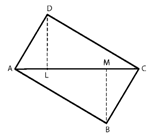Solution 7:

AC = 84 cm, DL = 16.5 cm and BM = 12 cm

Formula of the area of triangle =  x base x height

Area of triangle ADC =  1/2(AC x DL)

= 1/2 (84 x 16.5)

= 693 cm2

Area of triangle ABC =  1/2(AC x BM)

= 1/2(84 x 12) = 504 cm2

So, Area of quadrilateral ABCD = Area of triangle ADC + Area of triangle ABC

= (693 + 504) cm2

= 1197 cm2

Question :8. Find the area of the quadrilateral ABCD given in Fig. 30. The diagonals AC and BD measure 48 m and 32 m respectively and are perpendicular to each other.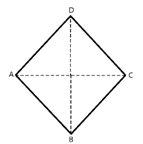Solution  8:

Diagonal AC = 48 m and diagonal BD = 32 m
Formula of the area of a quadrilateral = 1/2 (Product of diagonals)
= 1/2 (AC x BD)
= 1/2 (48 x 32) m2
= 768 m2

Question :9.  In Fig 31, ABCD is a rectangle with dimensions 32 m by 18 m. ADE is a triangle such that EF⊥ AD and EF= 14 cm. Calculate the area of the shaded region.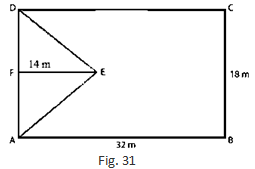Solution 9:

Length of rectangle = 32m and breadth of rectangle = 18m

Formula of the area of rectangle = length x breadth

Thus, area of the rectangle = AB x BC

= 32 m x 18 m

= 576 m2

base of triangle = 18m and height of triangle

Formula of the area of triangle =  1/2x base x height

Area of the triangle =  (AD x FE)

= 1/2 (BC x FE) (As AD = BC)

=  1/2 (18 m x 14 m)

= 126 m2

Area of the shaded region = Area of the rectangle – Area of the triangle

= (576 – 126) m2

= 450 m2

Question :10. In Fig. 32, ABCD is a rectangle of length AB = 40 cm and breadth BC = 25 cm. If P, Q, R, S be the mid-points of the sides AB, BC, CD and DA respectively, find the area of the shaded region.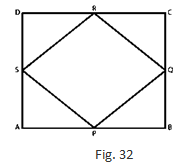Solution 10: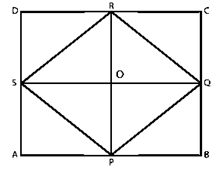ABCD is a rectangle of length AB = 40 cm and breadth BC = 25 cm.
Join PR and SQ so that these two lines bisect each other at point O
OP = OR = RP/2
= 25/2
= 12.5 cm
According to the given diagram,
Area of Triangle SPQ = Area of Triangle SRQ
So, area of the shaded region = 2 x (Area of SPQ)
= 2 x { 1/2 (SQ x OP)}
= 2 x {1/2 (40 x 12.5)}
= 500 cm2

Question :11. Calculate the area of the quadrilateral ABCD as shown in Fig.33, given that BD = 42 cm, AC = 28 cm, OD = 12 cm and AC ⊥ BO.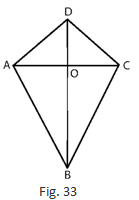Solution 11:

Given in the question BD = 42 cm, AC = 28 cm, OD= 12 cm

Formula of the area of Triangle ABC =  (AC x OB)

=  1/2(AC x (BD – OD))

=  1/2(28 cm x (42 cm – 12 cm))

=  1/2(28 cm x 30 cm)

= 14 cm x 30 cm

= 420 cm2

Area of Triangle ADC =  1/2(AC x OD) =  1/2(28 cm x 12 cm)

= 14 cm x 12 cm

= 168 cm2

So, Area of the quadrilateral ABCD = Area of triangle ABC + Area of triangle ADC

= (420 + 168) cm2

= 588 cm2

Question :12. Find the area of figure formed by a square of side 8 cm and an isosceles triangle with base as one side of the square and perimeter as 18 cm.

Solution  12:Let x cm be one of the equal sides of an isosceles triangle.
The perimeter of the isosceles triangle = 18 cm
Then, x + x + 8 =18
2x = (18 – 8) = 10 cm
2x = 10
x = 5 cm
value of x is 5 cm
Area of the diagram formed = Area of the square + Area of the isosceles triangle
= (side of square)2 + ½ (base x √[(equal side)2 – ¼ x (base)2
= 82 + ½ (8) x √[52 – 1/4 x 82
= 64 + 4 x √[25 – 1/4 x 64]

= 64 + 4 x √(25 – 16)

= 64 + 4 x √9
= 64 + 4 x 3
= 64 + 12
= 76 cm2

Question :13. Find the area of Fig. 34, in the following ways: (i) Sum of the areas of three triangles (ii) Area of a rectangle — sum of the areas of five triangles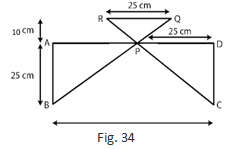Solution 13:

(i) According to the figure, Midpoint of AD is P.

Thus AP = PD = 25 cm and AB = CD = 20 cm

According to the figure,

Area of Triangle APB = Area of Triangle PDC

Area of Triangle APB =  1/2(AB x AP)

= 1/2 (20 x 25)

= 250 cm2

Area of Triangle PDC = Area of Triangle APB = 250 c m2

Area of Triangle RPQ = ½ (Base x Height)

=  1/2(25 cm x 10 cm)

= 125 cm2

So, total of the three triangles are (250 + 250 + 125) cm2

= 625 cm2

(ii) According to the figure, area of the rectangle ABCD = 50 cm x 20 cm

= 1000 cm2

Thus, Area of the rectangle – Sum of the areas of three triangles

= (1000 – 625) cm2

= 375 cm2

Question :14. Calculate the area of quadrilateral field ABCD as shown in Fig.35, by dividing it into a rectangle and a triangle.Solution 14:Join CE, so that which intersect AD at point E.

AE = ED = BC = 25 m and EC = AB = 30 m

Formula of the area of rectangle = length x breadth

Area of the rectangle ABCE = AB x BC

= 30 m x 25 m

= 750 m2

Formula of the area of triangle = 1/2 x base x height

Area of Triangle CED =  1/2(EC x ED)

=  1/2(30 m x 25 m)

= 375 m2

So, Area of the quadrilateral ABCD = (750 + 375) m2 = 1125 m2

Question :15. Calculate the area of the pentagon ABCDE, where AB = AE and with dimensions as shown in Fig. 36.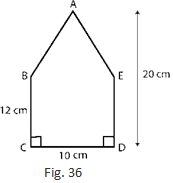Solution 15: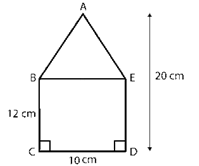Join BE so that we can get rectangle and triangle.

Formula of the area of rectangle = length x breadth

Area of the rectangle BCDE = 1/2(CD x DE)

= 10 cm x 12 cm

= 120 c m2

Formula of the area of triangle = 1/2 x base x height

Area of Triangle ABE =  1/2(BE x height of the triangle)

=  1/2(10 cm x (20 – 12) cm)

= 1/2(10 cm x 8 cm)

= 40 cm2

So, Area of the pentagon ABCDE = area of rectangle + area of triangle

= (120 + 40) cm2

= 160 cm2

Question :16. The base of a triangular field is three times its altitude. If the cost of cultivating the field at Rs 24.60 per hectare is Rs 332.10, find its base and height.

Solution  16:

Let be h m altitude of the triangular field.

Then base of the triangular field is 3h m.

Formula of the area of triangle =  1/2 x b x h

Area of the triangular field = 1/2(h x 3h) = 3h2/2 m2 - (i)

The rate of cultivating the field is Rs 24.60 per hectare.

Therefore,

Area of the triangular field =  332.10/24.60

= 13.5 hectare

= 135000 m2 (As 1 hectare = 10000 m2)- (ii)

By equation (i) and (ii),

3h2 /2= 135000 m2

3h2 = 135000 x 2 = 270000 m2

h2 = 270000/3

= 90000 m2

= (300)2

h = 300 m

So, Height of the triangular field = 300 m and

Base of the triangular field = 3 x 300 m = 900 m

Question :17. A wall is 4.5 m long and 3 m high. It has two equal windows, each having form and dimensions as shown in Fig. 37. Find the cost of painting the wall (leaving windows) at the rate of Rs 15 per m2.Solution 17:

Length of a wall = 4.5 m and breadth of the wall = 3 m

Formula of the area of triangle = length x Breadth

Area of the wall = Length x Breadth

= 4.5 m x 3 m = 13.5 m2

According to the diagram,

Area of the window = Area of the rectangle + Area of the triangle

= (0.8 m x 0.5 m) + ( 1/2 x 0.8 m x 0.2 m)                      (As 1 m = 100 cm)

= 0.4 m2 + 0.08 m2

= 0.48 m2

Area of two windows = 2 x 0.48 = 0.96 m2

Area of the remaining wall (leaving windows) = (13.5 – 0.96) m2

= 12.54 m2

price of painting the wall per m2 = Rs. 15

So, the price of painting the wall = Rs. (15 x 12.54)

= Rs. 188.1